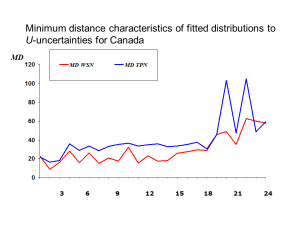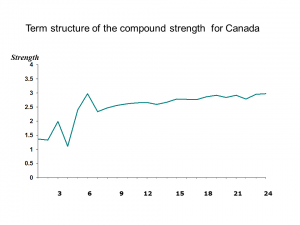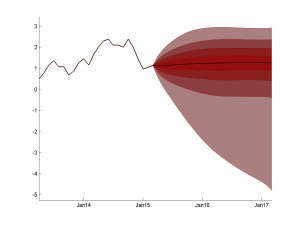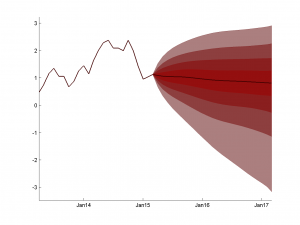# Probabilistic Approach to Assessing Macroeconomic Uncertainties

PRAMU

## ESRC/ORA Project

1. Figure 1. Minimum distance characteristics of fitted TPN and WSN distributions to U-uncertaintiesWSN fits better than TPN, especially for short and medium horizons.

2. Figure 2. Term structure of the compound strength of forecast-induced monetary policyThe compound strength is computed as: , where . In the policy-input symmetric case, where  (and this is the assumption applied for empirical estimation), the multipliers  can be ignored.

3. Table 1. Forecast uncertainty measures

4. Figure 3. Ex-post and ex-ante inflation fan chartsEx-ante uncertainty Ex-post uncertainty